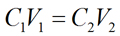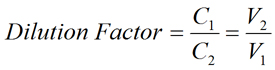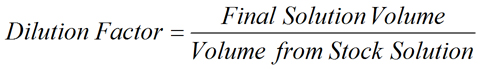PhysiologyWeb  Home  |  FAQ  |  About  |  Contact  |   - Sign InDilution Factor Calculator - No Unit
Meant to be used in both the teaching and research laboratory, this calculator (see below) can be utilized to perform dilution factor calculations. When a concentrated solution is diluted, the dilution factor may be expressed as the ratio of the concentration of stock solution to the concentration of the diluted solution. The dilution factor may also be expressed as the ratio of the volume of the final diluted solution to the initial volume removed from the stock solution. See below for the dilution factor equation.
For example, if 100 mL of a stock solution is diluted with solvent/diluent to a total, final volume of 1000 mL, the resulting dilution factor is 10. For this particular dilution, it may also be said that the stock solution was diluted 10-fold. As another example, a 2-fold dilution is the same as a dilution factor of 2. Therefore, dilution by any factor X is equivalent to X-fold dilution.
Please note that the dilution factor calculator below uses the metric unit for volume (fL, pL, nL, μL, mL, and L). If you wish to convert these units to other volume units, please use our Unit Conversion Calculator.
Additional dilution factor calculators are also available and are suited to more specialized applications (see here).
Dilution factor equation - no unit• Dilution Factor is the factor by which the stock solution is diluted. It may be expressed as the ratio of the volume of the final diluted solution (V2) to the initial volume removed from the stock solution (V1), as shown in the equation above. Dilution factor may also be expressed as the ratio of the concentration of stock solution (C1) to the concentration of the diluted solution (C2).
• Volume from Stock Solution (V1) is the volume to be removed (i.e., aliquoted) from the concentrated stock solution.
• Final Solution Volume (V2) is the final volume of the diluted solution. This is the volume that results after the volume from the stock solution (V1) has been diluted with solvent or diluent to achieve a total diluted volume of the final solution (V2). Please note that the final volume refers to the total solution volume, which is the combined volume of the stock solution and the volume of solvent/diluent used for dilution.
Dilution factor calculator - no unit
Each calculator cell shown below corresponds to a term in the formula presented above. Enter appropriate values in all cells except the one you wish to calculate. Therefore, at least two cells must have values, and no more than one cell may be blank. The value of the blank cell will be calculated based on the other values entered. After a calculation is performed, the calculated cell will be highlighted and subsequent calculations will calculate the value of the highlighted cell (with no requirement to have a blank cell). However, a blank cell has priority over a highlighted cell.
For convenience, this calculator allows you to select different volume units, and the necessary conversions are carried out for you to obtain the value of the blank cell in the desired unit.
 Dilution factor Volume from stock mL fL pL nL μL mL L Final solution volume mL fL pL nL μL mL L